# Rhombohedrons

To tile space with polyhedrons sharing faces, the sum of the solid angles at a common vertex must be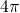. At each vertex O of a rhombohedron three rhomb faces meet. The solid angle at the vertex is the so-called excess or

(1)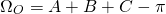The angles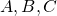are dihedral angles of pairs of faces

An isohedral or trigonal rhombohedron has two types of solid angles, two at the vertices on the trigonal axis and six at the other corners. The relationship between the opening angle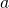of the rhomb and the dihedral angle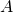is

(2)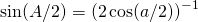If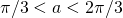there is also an obtuse rhombohedron and because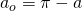(3)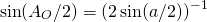There are a number of special trigonal rhombohedra, the cube (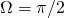), the acute and obtuse golden rhombohedra (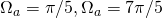) and the trigonal rhombohedron into which a regular rhombic dodecahedron can be decomposed (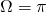).

The faces of the the golden rhombohedron are called golden rhombs, because the ratio of the lengths of its diagonals is the golden ratio. The opening angle of the rhomb is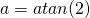. (Note that the golden rhombohedron differs from the Penrose rhomb.) Let the solid angles of an acute trigonal rhombohedron be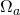and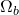and those of an obtuse trigonal rhombohedron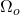and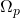. Their relationship isand. Therefore,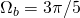and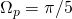. Two acute and two obtuse golden rhombohedrons can be combined to build an isohedral rhombic dodecahedron different from the regular rhombic dodecahedron. Furthermore, the golden rhombohedra are known to tile space aperiodically if certain matching rules are applied.